# Case Based Questions Test: Arithmetic Progressions

## 15 Questions MCQ Test NCERT Mathematics for CAT Preparation | Case Based Questions Test: Arithmetic Progressions

Description
Attempt Case Based Questions Test: Arithmetic Progressions | 15 questions in 30 minutes | Mock test for Class 10 preparation | Free important questions MCQ to study NCERT Mathematics for CAT Preparation for Class 10 Exam | Download free PDF with solutions
QUESTION: 1

### Read the following text and answer the following questions on the basis of the same: Amit has a packet of Candies. It consists of 288 candies. He arranges the candies in a way that first row contains 3 candies, second row has 5 and third row has 7 and so on.Q. How many candies are there in the last row?

Solution: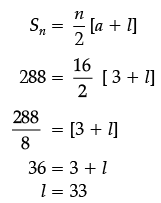QUESTION: 2

### Read the following text and answer the following questions on the basis of the same: Amit has a packet of Candies. It consists of 288 candies. He arranges the candies in a way that first row contains 3 candies, second row has 5 and third row has 7 and so on.Q. If Amit want to make 12 rows, then how many total candies will be placed by him with same arrangement.

Solution: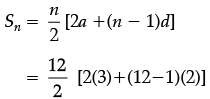= 6 [6 + 22]

= 148

QUESTION: 3

### Read the following text and answer the following questions on the basis of the same: Amit has a packet of Candies. It consists of 288 candies. He arranges the candies in a way that first row contains 3 candies, second row has 5 and third row has 7 and so on.Q. How many rows are there?

Solution: The A.P. is 3, 5, 7, ................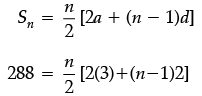⇒ 288 = n [3+(n−1)]

⇒ 288 = n2 + 2n

⇒ n2 + 2n − 288 = 0

⇒ n2 + 18n − 16n − 288 = 0

⇒ n(n + 18) − 16(n + 18) = 0

⇒ (n + 18)(n − 16) = 0

⇒ n = 16

QUESTION: 4

Read the following text and answer the following questions on the basis of the same: Amit has a packet of Candies. It consists of 288 candies. He arranges the candies in a way that first row contains 3 candies, second row has 5 and third row has 7 and so on.

Q. Find the difference in the candies placed in the 10th and 15th row.

Solution: a15 − a10 = a + 14d − a − 9d = 5d = 5(2) = 10
QUESTION: 5

Read the following text and answer the following questions on the basis of the same: Amit has a packet of Candies. It consists of 288 candies. He arranges the candies in a way that first row contains 3 candies, second row has 5 and third row has 7 and so on.

Q. Is there any row which contains 28 candies?

Solution: No, this is not possible if we see rows are having only odd number of candies.
QUESTION: 6

Read the following text and answer the following questions on the basis of the same: In a potato race, a bucket is placed at the starting point, which is 5 m from the first potato and the other potatoes are placed 3 m apart in a straight line. There are ten potatoes in the line (see figures).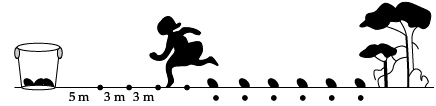A competitor starts from the bucket, picks up the nearest potato, runs back with it, drops it in the bucket, runs back to pick up the next potato, runs to the bucket to drop it in, and she continues in the same way until all the potatoes are dropped in the bucket.

Q. What is the distance run to pick up the 2nd potato?
Solution: Distance run to pick up the 2nd potato = 2 × (5 + 3) = 16 m
QUESTION: 7

Read the following text and answer the following questions on the basis of the same: In a potato race, a bucket is placed at the starting point, which is 5 m from the first potato and the other potatoes are placed 3 m apart in a straight line. There are ten potatoes in the line (see figures).A competitor starts from the bucket, picks up the nearest potato, runs back with it, drops it in the bucket, runs back to pick up the next potato, runs to the bucket to drop it in, and she continues in the same way until all the potatoes are dropped in the bucket.

Q. What is the distance run to pick up the 4th potato?

Solution: Distance run to pick up the

4th potato = 2 × (5 + 3 + 3 + 3)

= 28 m.

QUESTION: 8

Read the following text and answer the following questions on the basis of the same: In a potato race, a bucket is placed at the starting point, which is 5 m from the first potato and the other potatoes are placed 3 m apart in a straight line. There are ten potatoes in the line (see figures).A competitor starts from the bucket, picks up the nearest potato, runs back with it, drops it in the bucket, runs back to pick up the next potato, runs to the bucket to drop it in, and she continues in the same way until all the potatoes are dropped in the bucket.

Q. What is distance run to pick up the 1st potato?

Solution: Distance run to pick up the 1st potato = 2 × 5 = 10 m
QUESTION: 9

Read the following text and answer the following questions on the basis of the same: In a potato race, a bucket is placed at the starting point, which is 5 m from the first potato and the other potatoes are placed 3 m apart in a straight line. There are ten potatoes in the line (see figures).A competitor starts from the bucket, picks up the nearest potato, runs back with it, drops it in the bucket, runs back to pick up the next potato, runs to the bucket to drop it in, and she continues in the same way until all the potatoes are dropped in the bucket.

Q. What is the distance run to pick up the 3rd potato?
Solution: Distance run to pick up the 3rd potato = 2 × (5 + 3 + 3) = 22 m.
QUESTION: 10

Read the following text and answer the following questions on the basis of the same: In a potato race, a bucket is placed at the starting point, which is 5 m from the first potato and the other potatoes are placed 3 m apart in a straight line. There are ten potatoes in the line (see figures).A competitor starts from the bucket, picks up the nearest potato, runs back with it, drops it in the bucket, runs back to pick up the next potato, runs to the bucket to drop it in, and she continues in the same way until all the potatoes are dropped in the bucket.

Q. What is the total distance run by the competitor?
Solution: The sequence of distances is 10, 16, 22, 28.

a2 – a1 = 16 – 10 = 6

a3 – a2 = 22 – 16 = 6

a4 – a3 = 28 – 22 = 6

a2 – a1 = a3 – a2 = a2 – a1

∴ The sequence forms an A.P.

Here a = 10, d = a2 – a1= 16 – 10 = 6, n = 10

Now, total distance run by competitor = S10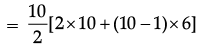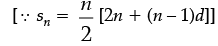= 5[20 + 9 × 6]

= 5[20 + 54]

= 5 × 74

= 370 m

QUESTION: 11

A manufacturer of TV sets produced 600 sets in the third year and 700 sets in the seventh year, assuming that the production increases uniformly by a fixed number every year.

Q. Find the production in the 10th year.

Solution: a10 = a + 9d = 550 + 9(25) = 550 + 225 = 775
QUESTION: 12

A manufacturer of TV sets produced 600 sets in the third year and 700 sets in the seventh year, assuming that the production increases uniformly by a fixed number every year.

Q. What is the difference between the productions of two consecutive years?

Solution: The difference between the productions of two consecutive years is 25.
QUESTION: 13

A manufacturer of TV sets produced 600 sets in the third year and 700 sets in the seventh year, assuming that the production increases uniformly by a fixed number every year.

Q. Find the production in the first year?

Solution: Let a be the production in first year and d be the difference between the productions of two consecutive years.

a3 = a + 2d = 600 …(i)

a7 = a + 6d = 700 …(ii)

Subtracting (i) from (ii),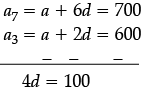d = 25

Put value of d in (i), we get

a = 550

QUESTION: 14

A manufacturer of TV sets produced 600 sets in the third year and 700 sets in the seventh year, assuming that the production increases uniformly by a fixed number every year.

Q. Find the total production in the first 7 years.

Solution: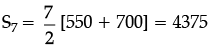QUESTION: 15

A manufacturer of TV sets produced 600 sets in the third year and 700 sets in the seventh year, assuming that the production increases uniformly by a fixed number every year.

Q. If they produced 600 sets in the year 2010. Then in what years their production will be 1000?

Solution: an = a + (n – 1)d

1000 = 600 +(n – 1) 25

400 = (n – 1) 25

16 = (n – 1)

17 = n

Therefore, in the year 2027.Use Code STAYHOME200 and get INR 200 additional OFF Use Coupon Code